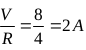# Qn) A student was asked to compare the resistance of two conductors using a meter bridge apparatus. a) What is the working principle of Meter Bridge. b) Draw the necessary circuit diagram for the experiment. c) Write an expression for the ratio of resistances. d)A Meter bridge wire has resistance0.2 Ω /cm. The bridge is balanced at 60cm from the end of an unknown resistance X. If the known resistance is 2 Ω , find the current flowing through the circuit in the balanced condition if a cell of 8V is connected in the circuit.

a) Wheatstone’s Principle

b)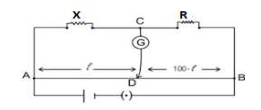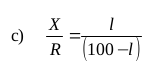d)When the bridge is balanced R AD , R DB of the meter bridge are in series. Also R and X are
in series.
Effective resistance of R AD and R DB = 100×0.2 =20Ω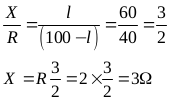Effective resistance of Rand X in series = R + X = 3+2=5Ω

Effective resistance of the network =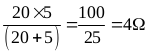Current through the circuit I=